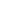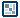## Question of the WeekSprings: Elasticity, Physics, and Flash MX          written by ilyas usal a.k.a. pom In this tutorial, we will see how to give an object an effect of elasticity. The physics behind it: We will take the example of a mass attached to a spring. Let's say that the normal state of the system, that is to say when nothing moves, in other words the equilibrium, happens when the spring has a length l. If you move the mass by dx, making so that the spring has now a length l', the force will then be   -k.dx = -k(l'-l) k is a specific to each spring, and it is <1. All this to show you what you already know : the more we pull on the spring, the faster it will go. Here, pulling hard means big dx, hence a big force, and a high speed.   But we must not forget about inertia. The mass always tends to go back to equilibrium, but it has a certain speed when it does, so it doesn't just stop there. Let's say now that we want the mass to oscillate around the mouse position. In Flash we'll translate this like this : onClipEvent (load) { // inertia relates to the quantity of energy that // the spring will carry // inertia = 1 would mean that the spring doesn't // loose any energy, and that it will oscillate // forever inertia = 0.9 ; // k relates to the spring, and how "hard" it will be. // The higher k the faster the mass will come back. k = 0.1 ; } onClipEvent (enterFrame) { // We calculate the distance to the mouse x = -this._x + _root._xmouse ; y = -this._y + _root._ymouse ; //We calculate the amount by which the mass will to move xp = xp * inertia + x*k ; yp = yp * inertia + y*k ; //We move it _x += xp ; _y += yp ; }   This code goes inside the Actions panel of the mass, which has to be a movie clip. To make the code more reusable, we are going to define a prototype that will do the job for us. Basically, it's just a function that any movie clip can call from anywhere. In the main scene, create a new layer, and call it code. Select the first frame, and open the Frame Actions panel. MovieClip.prototype.move = function (centerx,centery,inertia,k) { x = -this._x + centerx ; y = -this._y + centery ; xp = xp * inertia + x*k ; yp = yp * inertia + y*k ; _x += xp ; _y += yp ; } This replaces the code the movie clip had. onClipEvent (enterFrame) { this.move (_root._xmouse,_root._ymouse,0.9,0.1) ; }   [ The following works only with Flash 5. I'll have to find out a way with Flash MX ] If you put 3 movie clips on the stage, you can very easily give them different elastic effects by changing the parameters inertia and k. Here's an example: Now imagine that we want to drag the mass and let it go. The first thing we have to do is check if the mass is being dragged. In order to do so, we'll put a button inside the mass movie clip, with the code : on (press) { pressing = 1 ; } on (release) { pressing = 0 ; } If we're not pressing, we will execute the prototype move, but if we're pressing, we have to start dragging the movie clip. Hence the code : onClipEvent (load) { // We define the reference point centerx = 100 ; centery = 100 ; } onClipEvent (enterFrame) { if (pressing) startDrag (this,true) ; else { stopDrag () ; this.move (centerx,centery,0.9,0.1) ; } } The animation after the code has been input: To make the effect really realistic, we have to make so that if we give it rotation, it keeps the rotation when we let it go. We need to modify the previous code slightly: onClipEvent (load) { // We define the reference point centerx = 100 ; centery = 100 ; // temporary variables used to calculate // the speed of the mass x0 = this._x ; y0 = this._y ; } onClipEvent (enterFrame) { if (pressing) { startDrag (this,true) ; xp = this._x - x0 ; yp = this._y - y0 ; x0 = this._x ; y0 = this._y ; } else { stopDrag () ; this.move (centerx,centery,0.9,0.1) ; } } The final animation after the code has been replaced with the above code: Click here for all of the source files for this tutorial on springs and making them work elastically. This tutorial is written by Ilyas Usal. Ilyas is also known as ilyaslamasse on the kirupa.com forums! If you have any questions, please post them on the forums by clicking here.   pom